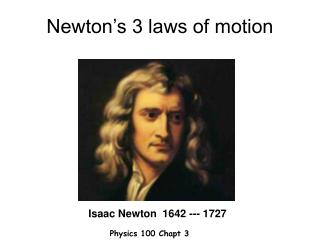# Newton’s 3 laws of motion - PowerPoint PPT PresentationDownload PresentationNewton’s 3 laws of motion

Newton’s 3 laws of motionDownload Presentation## Newton’s 3 laws of motion

- - - - - - - - - - - - - - - - - - - - - - - - - - - E N D - - - - - - - - - - - - - - - - - - - - - - - - - - -
##### Presentation Transcript

1. Newton’s 3 laws of motion Isaac Newton 1642 --- 1727 Physics 100 Chapt 3

2. 1st Law: Law of Inertia A body at rest tends to stay at rest, a body in motion tends to keep moving along at a constant speed and in a straight-line path unless interfered with by some external forces.

3. example

4. Motorcycle crash dummy

6. 2nd Law: F=ma The acceleration of a body is directly proportional to the netforce acting on it and inversely proportional to its mass.The direction of the acceleration is in the direction of the applied force.

7. Directly proportional to Force a a Small force Small acceleration Large force Largeacceleration

8. inversely proportional to mass a a Beach ball Bowling ball small mass Large acceleration Large mass Smallacceleration

9. “Inertial” mass “Inertial” mass, mi, is the resistance to changes in the state of motion Objects with large mi are hard to get moving (& once started, hard to stop), Objects with small mi easier to get moving (& easier to stop),

10. Units of mass (we cant avoid them!) Mass: basic unit = 1kilogram = 1kg mass of 1 liter of water 10cm This much water! 10cm 10cm

11. Net force Tip-to-tail method for adding vector Net force is the vector from the tail of the 1st to the tip of the 2nd. (0 in this case). Slide tail of one to tip of the other (keep directions fixed)

12. Tip-to-tail method Net forcepoints down the hill Slide tail of one to tip of the other (keep directions fixed)

13. Newton’s 2nd law  F=ma a is proportional to F: a  F direction of a = direction of F: a  F a is inversely proportional to m: a  1/m combine: a  F/m multiply both sides by m set proportionality constant = 1: a = F/m

14. Units of Force F=ma m s2 kg Unit of force: 1 Newton = 1N = 1 kg m/s2 1 pound =1lb = 4.5 N

15. What is your mass? Suppose I jump off a tqble Weight = force of Earth’s gravity on you F=ma W=mg a=g m= W g W

16. Two different aspects of mass Force of gravity is proportional to “gravitational” mass mgg Weight: W = mg Newton’s 2nd law: Inertia; resistance to changes in state is proportional to “inertial” mass F m a = mi Experiment shows: mg = mi

17. !!!!! My mass My weight in pounds: W = 190 lbs Convert to Newtons: W = 190 lbs x 4.5 N/lb = 855 N 855 N 10 m/s2 m = W/g = = 85.5 kg

18. Newton’3rd Law: action-reaction Whenever one object exerts a force on a second object, the second object exerts an equal in magnitude but opposite in direction force on the first. reaction: the canoe pushes me forward action: I push on the canoe

19. Action Reaction

20. I push on the bus v= 0 F

21. But I accelerate v Newton: The bus exerted an “equal but opposite” force on me.

22. Look again All forces come in pairs! -F F This force causes me to accelerate backwards This force tries to accel. the bus forward

23. Air-filled balloon reaction: air pushes on balloon action: balloon pushes on air

24. recoil action: gun exerts force F2 on bullet making it accelerate reaction: equal but opposite force on the gun F1 Produces a recoil

25. Rocket propulsion reaction: rocket gets pushed in the opposite direction action: rocket engine pushes exhaust gasses out the rear